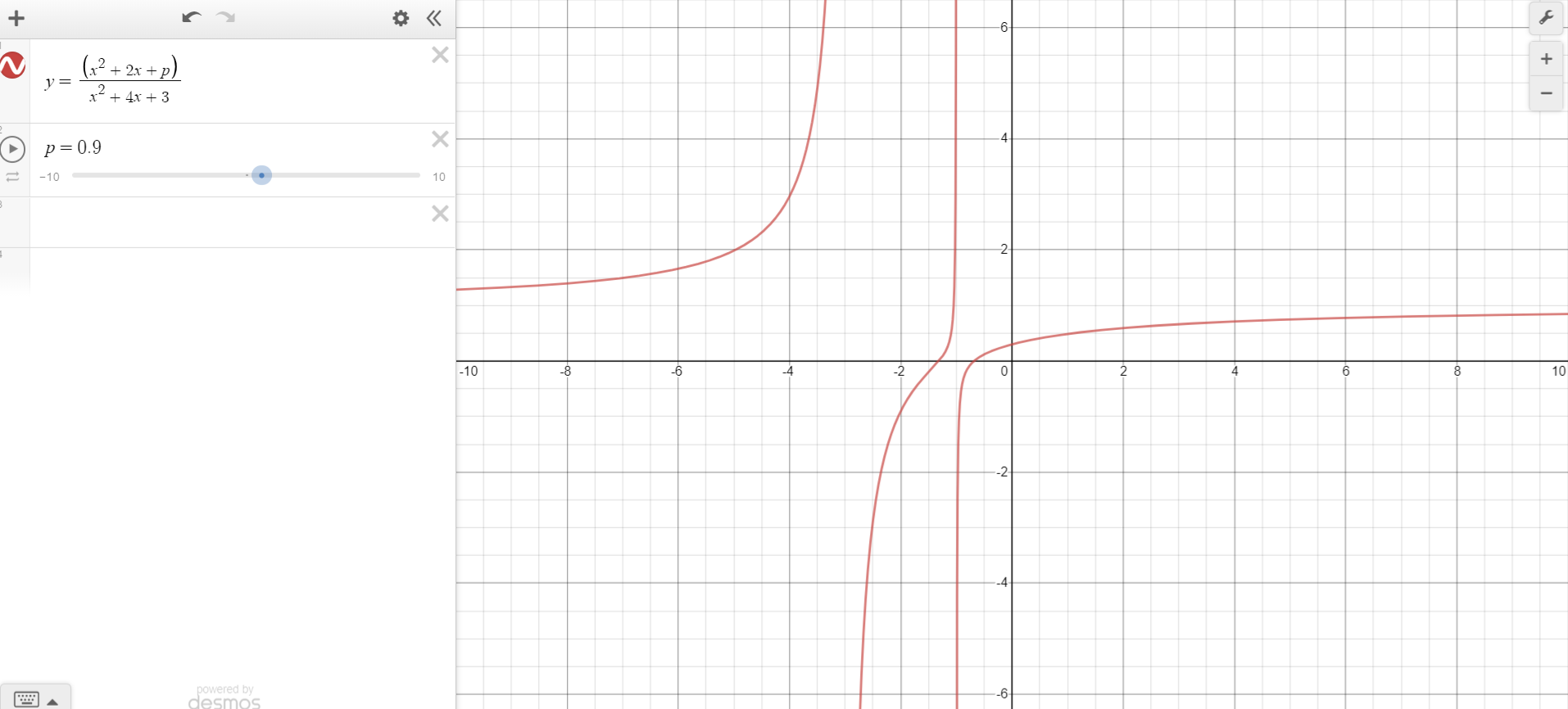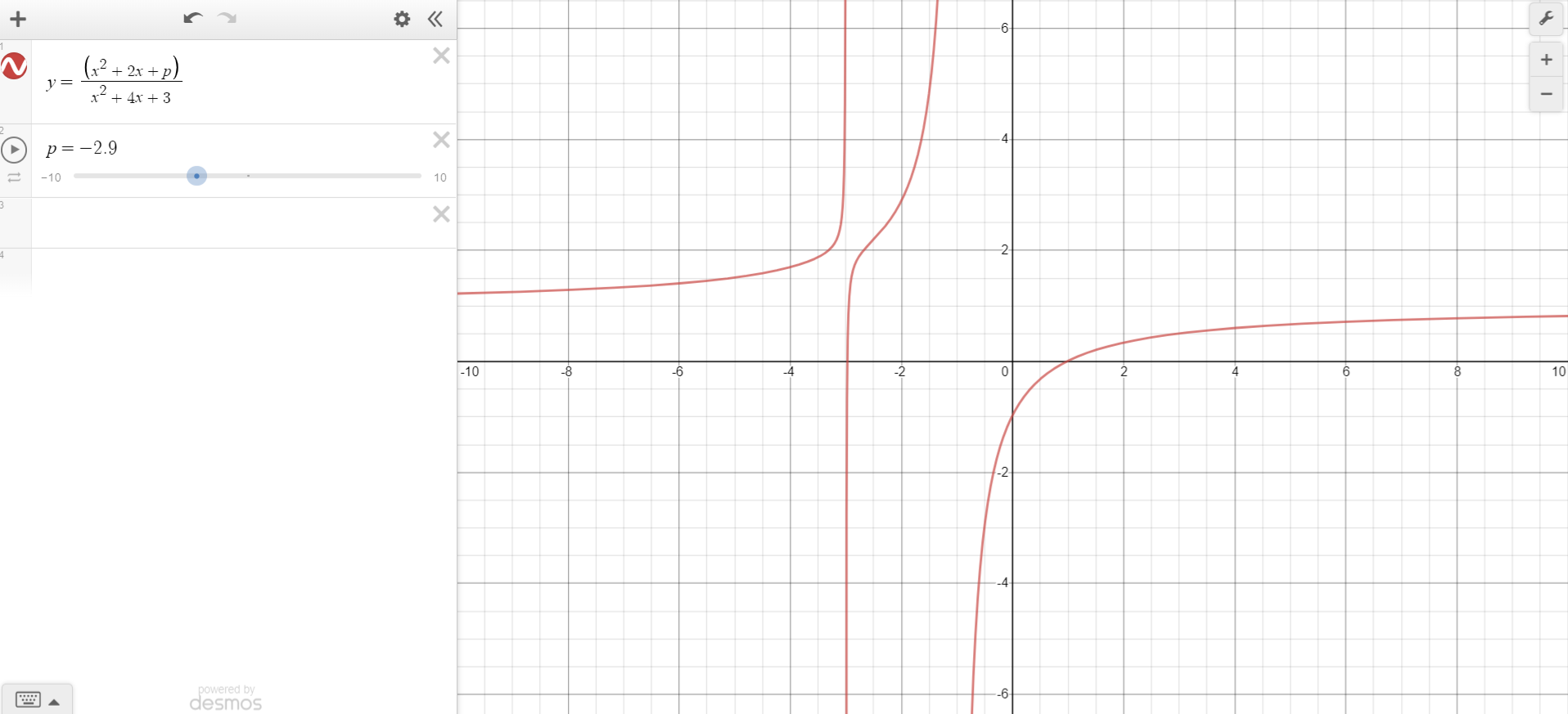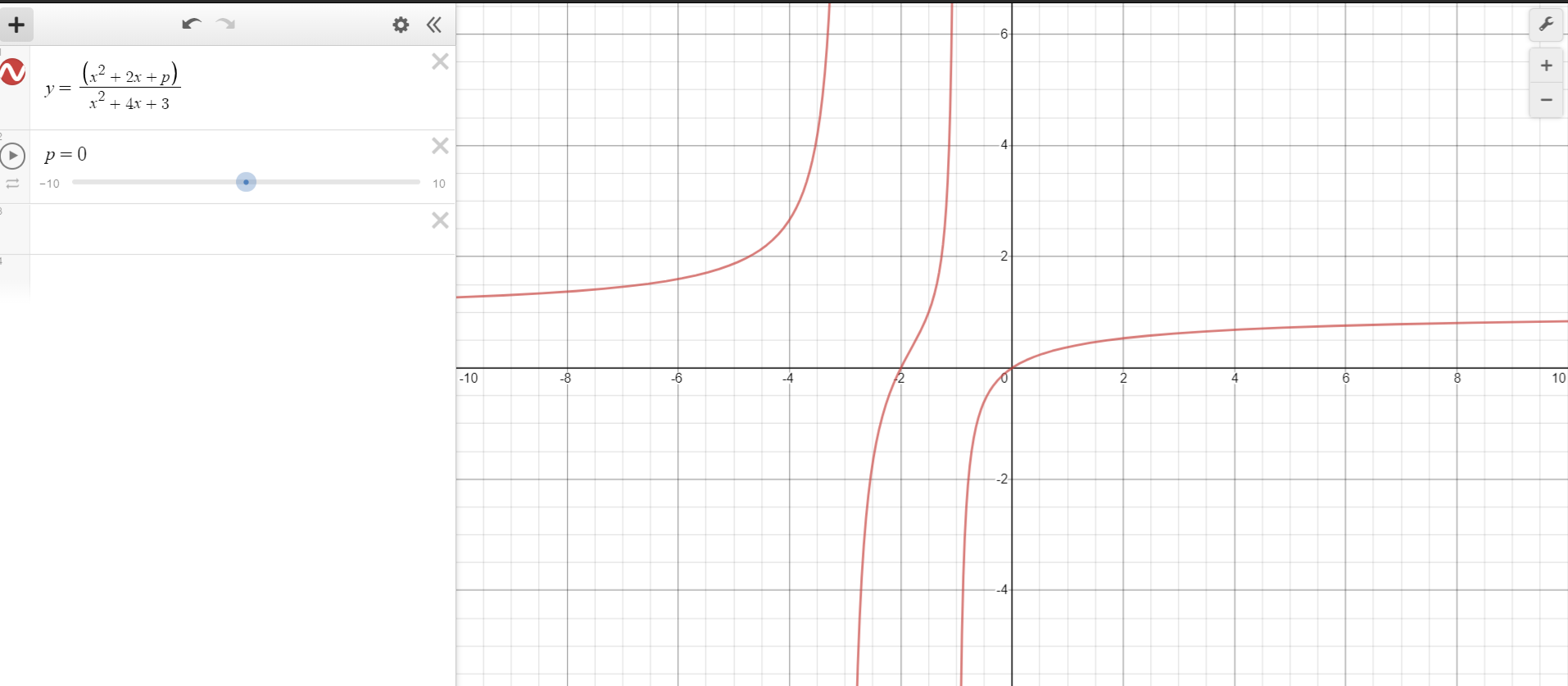I was solving some questions related to Quadratic Equations from my book, but I was unable to solve this question. Any help will be greatly appreciated!

## Question

For all real values of $x$,

$\dfrac{x^2+2x+p}{x^2+4x+3}$ can take real value if:

$\text{(A)} 0

$\text{(B)} 0\leq p\leq 1$

$\text{(C)} -1

$\text{(D)} -3\leq p\leq 1$

$\text{(D)} -3\leq p\leq 1$Note by Vinayak Srivastava
6 months, 2 weeks ago

This discussion board is a place to discuss our Daily Challenges and the math and science related to those challenges. Explanations are more than just a solution — they should explain the steps and thinking strategies that you used to obtain the solution. Comments should further the discussion of math and science.

When posting on Brilliant:

• Use the emojis to react to an explanation, whether you're congratulating a job well done , or just really confused .
• Ask specific questions about the challenge or the steps in somebody's explanation. Well-posed questions can add a lot to the discussion, but posting "I don't understand!" doesn't help anyone.
• Try to contribute something new to the discussion, whether it is an extension, generalization or other idea related to the challenge.

MarkdownAppears as
*italics* or _italics_ italics
**bold** or __bold__ bold
- bulleted- list
• bulleted
• list
1. numbered2. list
1. numbered
2. list
Note: you must add a full line of space before and after lists for them to show up correctly
paragraph 1paragraph 2

paragraph 1

paragraph 2

[example link](https://brilliant.org)example link
> This is a quote
This is a quote
    # I indented these lines
# 4 spaces, and now they show
# up as a code block.

print "hello world"
# I indented these lines
# 4 spaces, and now they show
# up as a code block.

print "hello world"
MathAppears as
Remember to wrap math in $$ ... $$ or $ ... $ to ensure proper formatting.
2 \times 3 $2 \times 3$
2^{34} $2^{34}$
a_{i-1} $a_{i-1}$
\frac{2}{3} $\frac{2}{3}$
\sqrt{2} $\sqrt{2}$
\sum_{i=1}^3 $\sum_{i=1}^3$
\sin \theta $\sin \theta$
\boxed{123} $\boxed{123}$

Sort by:

Let $y=\dfrac{x^2+2x+p}{x^2+4x+3}$ Then $yx^2+4xy+3y=x^2+2x+p$ $yx^2-x^2+4xy-2x+3y-p=0$ $\implies (y-1)x^2+ (4y-2)x +3y-p=0$ Since $x$ is real, $D\geq0$ $(2y-1)^2-(3y-p)(y-1) \geq 0$ Solving, $y^2+(p-1)y-p+1 \geq 0$ Since $y$ is also real, here's $D$ is also $\geq 0$ $(p-1)^2 +4p-4 \geq 0$ Solving, $(p-1)(p+3)\geq 0$ $\therefore \boxed{p \in (-\infty,-3) \cup (1,\infty)}$

- 6 months, 2 weeks ago

This is confusing! You should start with $x^2+4x+3\neq 0$

- 6 months, 2 weeks ago

- 6 months, 2 weeks ago

The first to second step needs to include the condition “for $x \neq -3$ and $x \neq -1$”. This condition is never dealt with.

- 6 months, 2 weeks ago

I agree. If $x=-1$ or $x=-3$, then you're basically multiplying the equation by 0, which makes the entire working pointless.

- 6 months, 2 weeks ago

The problem asks for what values will the solutions be real. You did the right thing by finding the discrimnant but all you have to do is notice that

$If\quad (p-1)(p+3)\quad is\quad the\quad disrcrimnant\quad for\quad any\quad value\quad between\quad -3:\quad (-3+3)(-3-1)=0\quad (As\quad you\quad said\quad when\quad D>0\quad or\quad D=0\quad There\quad are\quad real\quad solutions)\quad or\quad between\quad 1:\quad (1-1)(1+3)=0\\ You\quad will\quad see\quad that\quad they\quad both\quad work\quad and\quad can\quad conclude\quad that\quad it\quad works\quad for\quad that\quad range.$

- 6 months, 1 week ago

Ok??? Use \ ( \ ) :)

- 6 months, 1 week ago

:) is only a happppppy bracket

- 6 months, 1 week ago

Thanks

- 6 months, 1 week ago

It looks like something is wrong with either the question or answer. Let's say that D is the answer. That means $p$ could be $0$, and according to the question, $f(x) = \frac{x^2 + 2x + 0}{x^2 + 4x + 3} = \frac{x(x + 2)}{(x + 1)(x + 3)}$ is a real value for all real values $x$. However, this is not true for $x = -1$ and $x = -3$ because $f(-1) = \frac{-1}{0}$ and $f(-3) = \frac{3}{0}$, neither of which are real numbers.

- 6 months, 2 weeks ago

I was also feeling like something is wrong. I asked someone, and they calculated that none of the options is true, instead, the answer is $p \in (-\infty,-3) \cup (1,\infty)$

- 6 months, 2 weeks ago

Hmmm... Your code is wrong :)

- 6 months, 2 weeks ago

Was wrong :)

- 6 months, 2 weeks ago

David Vreken is right. Test by plugging in some arbitrary values for $p$, say $p=524364536543543543$. You will still realize that the fraction $\frac{x^2+2x+p}{x^2+4x+3}$ does not always take real values. So the interval you've found/given/told is still wrong.

- 6 months, 2 weeks ago

Yeah! I can't understand the 0 denominator too! The problem is wrong. I think the solution is $(-\infty;-3)and(-3;-1)and(-1;\infty)$

- 6 months, 2 weeks ago

I agree that answer given is incorrect. Plus, I don't think so 0 is only one possible value of $p$. But, If I am not mistaken, you took $p = 0$ to prove something is flawed, right?

- 6 months, 2 weeks ago

But, what I did not understand was when he graphed the equation, D came out to be the answer! I don't know how??!!

- 6 months, 2 weeks ago

Can you take a photo about the solution? Or the solution isn't in english?

- 6 months, 2 weeks ago

"D" does not came out to be the answer. If it does, you should expect to see that it's continuous everywhere, which is obviously not true at the points $x=-1, -3$.

- 6 months, 2 weeks ago

When can a fraction give a non-real number, if the numerator and the denominator are real numbers?

- 6 months, 2 weeks ago

$\frac 00$.

- 6 months, 2 weeks ago

$\cfrac{0}{0}$ is undefinied. This can be 1 or 0.

- 6 months, 1 week ago

First, i ask what is $\frac{x}{0}$? if you say a value $k$ for instance either 1 or 0, then for every $x$, the values are equal. For example:

$\dfrac{4}{0} = k = \dfrac{9}{0} \implies \boxed{4 = 9}?$

- 6 months, 1 week ago

I learned like this at school: $\cfrac{n}{-0}=-\infty;\cfrac{n}{+0}=+\infty$ link to $\cfrac{0}{0}$

- 6 months, 1 week ago

That involves limits I guess. But there isn't a perfect definition for it, that's why it is undefined. You can get a very close approximation but the value of it might not be exact, you place a hollow circle on the graph for it. I think this where calculus comes in with determinate and indeterminate forms.

- 6 months, 1 week ago

I am writing what he wrote in my notebook, I don't fully understand, please wait! Thanks!

- 6 months, 2 weeks ago

However, the graph shows:The equation really has all values of $y$ in the range given in option $D$!!!!!!

- 6 months, 2 weeks ago

That's not correct. When $x = -1$ or when $x=-3$, the value of $y$ is undefined.

- 6 months, 2 weeks ago

# Even at p=0, y has all real values!- 6 months, 2 weeks ago

The question is not asking if the equation has all values of $y$ for a given range of $p$, it’s asking if there is a real value of $y$ for all values of $x$ for a given range of $p$. Unfortunately, for any value of $p$, there is at least one vertical asymptote (either at $x = -3$ or $x = -1$), so it’s not true for any given range or value of $p$.

- 6 months, 2 weeks ago

Also, something which I was thinking before I gave up:

$x^2+2x+p,$ needs to be real, so its discriminant is $\geq 0$

$(2)^2-4p \geq 0$ $\implies p\leq1$

- 6 months, 2 weeks ago

Yes, this is the basic solution. But you should give the intervals, where the fraction isn't real

- 6 months, 2 weeks ago

I have written the exact same words as in my book, I haven't changed even a single word of the question or options. So, if it is wrong, the book is wrong!

- 6 months, 2 weeks ago

This doesn't make sense. If $x^2 + 2x + p$ is real and $x$ is real, then trivially, $p$ must be real as well. We can't have $\text{real number} + \text{another real number} + \text{some non-real number} = \text{some real number}$.

- 6 months, 2 weeks ago

- 6 months, 2 weeks ago

So, from all the comments, I conclude that my book is wrong and the question was flawed. Thanks a lot @David Vreken, @Mahdi Raza, @Páll Márton for helping me!

- 6 months, 2 weeks ago

I don't see how this is possible. If the ratio of two polynomials with real coefficients is always real, then the denominator of this fraction must be non-zero for all $x$. Which means $x^2 + 4x + 3 \ne 0$ for all real $x$. But this is obviously false because $x=-1,-3$ makes the expression (the ratio of the two polynomials) undefined.

- 6 months, 2 weeks ago

Yes, thanks for replying. I am thinking of contacting the book authors to know what they thought while making the question, to know if there is some misprint. Thanks a lot @Pi Han Goh!

- 6 months, 2 weeks ago

No problem! =D

- 6 months, 2 weeks ago

@Pi Han Goh Now please post the solution of this Problem
I think may be, now you have deleted your solution, so wouldn't get another chance to upload solution.

- 6 months, 1 week ago